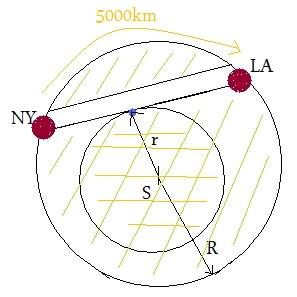# Tunnel between NY and LA

• onsem
In summary, an expert summarizer of content provided the following:-A tunnel is between Los Angeles and New York, and the distance between the two is 5000 kilometers.-The train moves in Simple Harmonic Motion with a period of 2πSQR(R/g) - the same as a low orbit satellite.-The gravitational field inside the earth is the same as if the mass was all at the center, and it is zero inside the shell.-If you let x be the displacement of the train from the middle of the tunnel, you find that the train's acceleration is -(g/R)x.-The gravity field inside the earth is the same as if the mass wasf

#### onsem

Hi,
I need help with interesting assigment.
So, here it is:
I have to work out time t. t is time of a trip by a tunnel digged between LA and NY. Distance between LA and NY by surface is 5000km. Earth radius is 6378km. Friction is ignored. The motion is in a train propeled by a gravitational force Fg. Earth is homogenous.
When the train is moveing towards the centre of Earth the radius is decreasing R->r and then after the centre is increasing again.

Picture for this assigment is here:
http://forum.matweb.cz/viewtopic.php?pid=51967#p51967

Thanks a lot.

This is an interesting and straight forward problem for the shorted route. A more interesting and very challenging problem is finding the fastest route. Almost certainly there is a parabolic tunnel that is faster. A parabolic tunnel will permit the train to accelerate faster at the beginning, with more rapid deceleration at the end. In about 1697, Isaac Newton was challenged to find the fastest route for a frictionless sliding mass to go from point x,y to a lower point x1,y1. He used his newly developed "Calculus of Variations" to solve the problem in one day (according to blogs). His solution, known as the "Brachistochrone Problem", can be found on the web. His solution assumes a constant g, which is not true in this tunnel problem, however. See
http://mathworld.wolfram.com/BrachistochroneProblem.html

I'm looking for solution only for this one case.Bob S might have said "cycloidal" rather than "parabolical" in his description
of the Brachistochrone problem.

Meantime, your diagram is particularly confusing.

You need to know that the gravity outside a spherical shell is
the same as if its mass was all at the centre, and that it is zero
inside the shell.

For a "homogeneous" Earth (constant density), gravity inside is gr/R.

If you let x be the displacement of the train from the middle of the tunnel,
you find that that the train's acceleration is -(g/R)x.

So no matter where the tunnel is drilled, the train moves in Simple Harmonic Motion
with period 2piSQR(R/g) - the same as a low orbit satellite!

David

Last edited:
Bob S might have said "cycloidal" rather than "parabolical" in his description
of the Brachistochrone problem.

Meantime, your diagram is particularly confusing.

You need to know that the gravity outside a spherical shell is
the same as if its mass was all at the centre, and that it is zero
inside the shell.

For a "homogeneous" Earth (constant density), gravity inside is gr/R.

If you let x be the displacement of the train from the middle of the tunnel,
you find that that the train's acceleration is -(g/R)x.

So no matter where the tunnel is drilled, the train moves in Simple Harmonic Motion
with period 2piSQR(R/g) - the same as a low orbit satellite!

David

How are you accounting for the angle of the incline in the application of gravity? It changes through out the tunnel, and is 90° and hence no contribution to motion in the middle.

I Don't get it.
what is that circle in the middle of the earth?
How much time does it take to travel from NY and LA by a straight tunnel inside the earth...
this can be done by simple harmonic motion ,or another way.
just tell me if this is the problem.
BTW for the problem I stated above, the time is constant for any distance you choose.

EDIT:
Just read David post, and he is right IMO, If this is the problem.
and the answer should be 42 minutes approx(way faster than an airplane :}).

Last edited:
How are you accounting for the angle of the incline in the application of gravity? It changes through out the tunnel, and is 90° and hence no contribution to motion in the middle.

sine of angle = x/r

gr/R * x/r = (g/R)*x

I agree a sensible diagram would help. Draw one:)

David

Ohhh, Now i get what is that circle in the sketch!
It's 2 show what David said, that the effective mass reduces and now the force is M=M(earth)*(r^3 / R(Earth)^3)/r^2 when r is smaller than R(Earth), inside earth.
which finally gives us g(inside Earth aka acceleration,towards the center )=g*x/R(earth) when x is the is the distance from the center of the earth.
i think :D